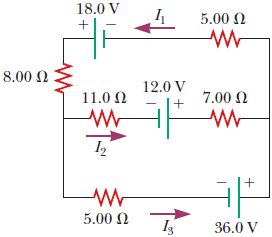# Problem: For the circuit shown in the figure below, we wish to find the currents I1, I2, and I3.(a) Use Kirchhoff's rules to complete the equation for the upper loop. (Use any variable or symbol stated above as necessary. All currents are given in amperes. Do not enter any units.)(b) Use Kirchhoff's rules to complete the equation for the lower loop. (Use any variable or symbol stated above as necessary. All currents are given in amperes. Do not enter any units.)(c) Use Kirchhoff's rules to obtain an equation for the junction on the left side. (Use any variable or symbol stated above as necessary. All currents are given in amperes.)(d) Solve the junction equation for I3.(e) Using the equation found in part (d), eliminate I3 from the equation found in part (b). (Use any variable or symbol stated above as necessary. All currents are given in amperes. Do not enter any units.)(f) Solve the equations found in part (a) and part (e) simultaneously for the two unknowns for I1 and I2, respectively.(g) Substitute the answers found in part (f) into the junction equation found in part (d), solving for I3.

###### FREE Expert Solution
100% (464 ratings)View Complete Written Solution
###### Problem Details

For the circuit shown in the figure below, we wish to find the currents I1, I2, and I3.(a) Use Kirchhoff's rules to complete the equation for the upper loop. (Use any variable or symbol stated above as necessary. All currents are given in amperes. Do not enter any units.)

(b) Use Kirchhoff's rules to complete the equation for the lower loop. (Use any variable or symbol stated above as necessary. All currents are given in amperes. Do not enter any units.)

(c) Use Kirchhoff's rules to obtain an equation for the junction on the left side. (Use any variable or symbol stated above as necessary. All currents are given in amperes.)

(d) Solve the junction equation for I3.

(e) Using the equation found in part (d), eliminate I3 from the equation found in part (b). (Use any variable or symbol stated above as necessary. All currents are given in amperes. Do not enter any units.)

(f) Solve the equations found in part (a) and part (e) simultaneously for the two unknowns for I1 and I2, respectively.

(g) Substitute the answers found in part (f) into the junction equation found in part (d), solving for I3.